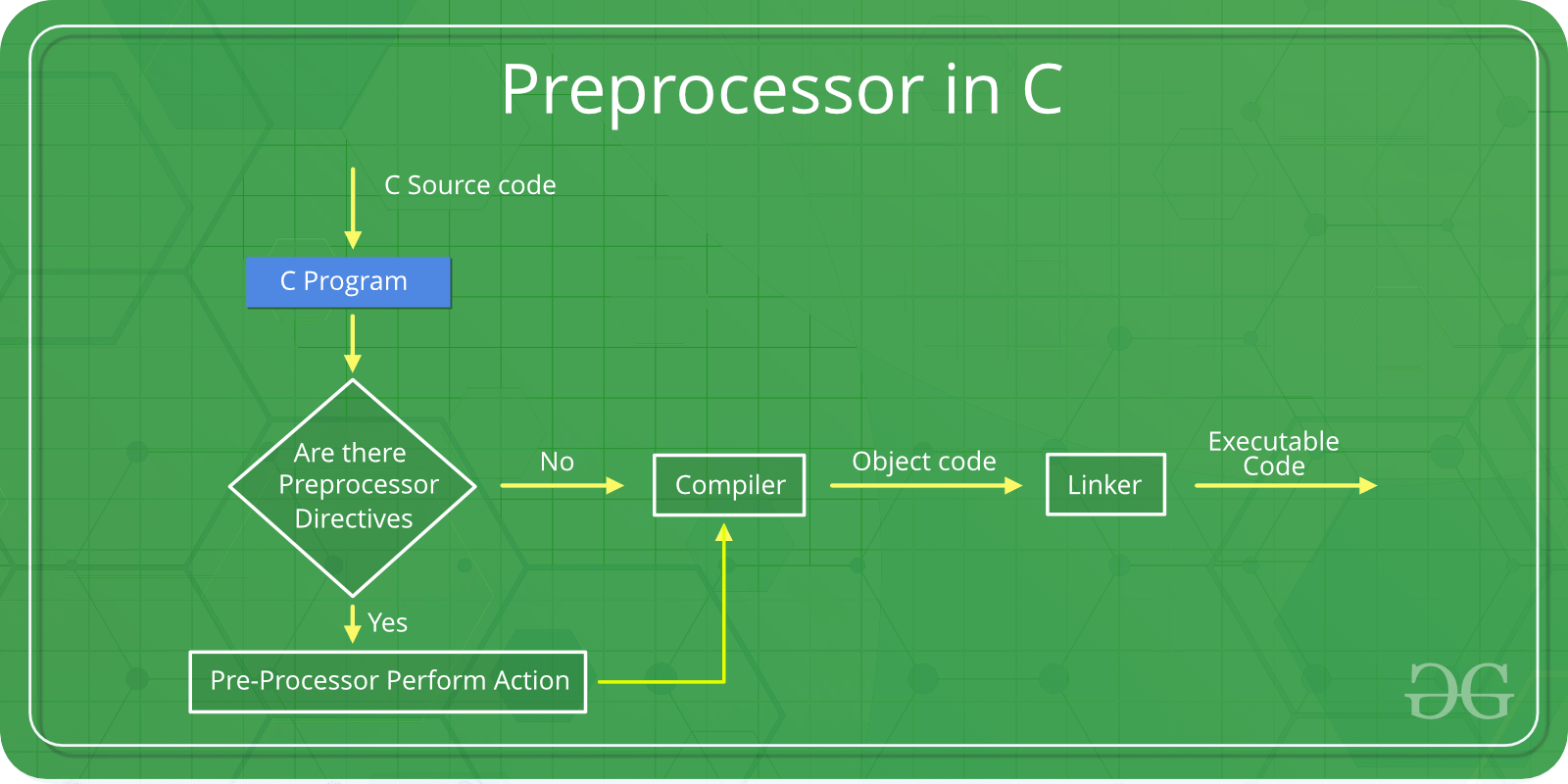# 1.2: Understanding Preprocessing

$$\newcommand{\vecs}{\overset { \rightharpoonup} {\mathbf{#1}} }$$ $$\newcommand{\vecd}{\overset{-\!-\!\rightharpoonup}{\vphantom{a}\smash {#1}}}$$$$\newcommand{\id}{\mathrm{id}}$$ $$\newcommand{\Span}{\mathrm{span}}$$ $$\newcommand{\kernel}{\mathrm{null}\,}$$ $$\newcommand{\range}{\mathrm{range}\,}$$ $$\newcommand{\RealPart}{\mathrm{Re}}$$ $$\newcommand{\ImaginaryPart}{\mathrm{Im}}$$ $$\newcommand{\Argument}{\mathrm{Arg}}$$ $$\newcommand{\norm}{\| #1 \|}$$ $$\newcommand{\inner}{\langle #1, #2 \rangle}$$ $$\newcommand{\Span}{\mathrm{span}}$$ $$\newcommand{\id}{\mathrm{id}}$$ $$\newcommand{\Span}{\mathrm{span}}$$ $$\newcommand{\kernel}{\mathrm{null}\,}$$ $$\newcommand{\range}{\mathrm{range}\,}$$ $$\newcommand{\RealPart}{\mathrm{Re}}$$ $$\newcommand{\ImaginaryPart}{\mathrm{Im}}$$ $$\newcommand{\Argument}{\mathrm{Arg}}$$ $$\newcommand{\norm}{\| #1 \|}$$ $$\newcommand{\inner}{\langle #1, #2 \rangle}$$ $$\newcommand{\Span}{\mathrm{span}}$$$$\newcommand{\AA}{\unicode[.8,0]{x212B}}$$

# C/C++ Preprocessors

As the name suggests Preprocessors are programs that process our source code before compilation. There are a number of steps involved between writing a program and executing a program in C / C++. Let us have a look at these steps before we actually start learning about Preprocessors.You can see the intermediate steps in the above diagram. The source code written by programmers is stored in the file program.c. This file is then processed by preprocessors and an expanded source code file is generated named program. This expanded file is compiled by the compiler and an object code file is generated named program .obj. Finally, the linker links this object code file to the object code of the library functions to generate the executable file program.exe.

Preprocessor programs provide preprocessors directives which tell the compiler to preprocess the source code before compiling. All of these preprocessor directives begin with a ‘#’ (hash) symbol. The ‘#’ symbol indicates that, whatever statement start with #, is going to preprocessor program, and preprocessor program will execute this statement. Examples of some preprocessor directives are: #include#define#ifndef etc. Remember that # symbol only provides a path that it will go to preprocessor, and command such as include is processed by preprocessor program. For example include will include extra code to your program. We can place these preprocessor directives anywhere in our program.

There are 4 main types of preprocessor directives:

1. Macros
2. File Inclusion
3. Conditional Compilation
4. Other directives

Let us take a brief look at each of these preprocessor directives.

### Macros

• In C++ macros are always proceeded by #define. Macros are a piece of code which is given some name. Whenever this name is encountered by the preprocessor the preprocessor replaces the name with the actual piece of code. The ‘#define’ directive is used to define a macro. Let us now understand the macro definition with the help of a program:
#include <iostream>
#define MAX 15

std::cout << "The max value is " << MAX << std::endl;

The word ‘MAX’ in the macro definition is called a macro template and ‘15’ is macro expansion. In the above program, when the pre-processor part of the compiler encounters the word MAX it replaces it with 15. It is as if you typed the code with the number 15 instead of the word MAX. The idea is that if you change your code, you only have to make a single edit, you do NOT have to go through your code and find every place where you have used that value. This may not seem like that big of a deal - until you have a coding project with 100,000 lines of code - you really don't want to sit and go through our code or even do a find-and-replace operation.
Note: There is no semi-colon(‘;’) at the end of macro definition. Macro definitions do not need a semi-colon to end.

Macros with arguments: We can also pass arguments to macros. Macros defined with arguments works similarly as functions. In the following example there are 2 macros - one for AREA which takes 2 arguments and multiplies them. The other macro is DOUBLE_IT, which takes 2 arguments and multiplies them together, but this macro is used to show how macros can look straight forward, but actually be broken.

#include <iostream>

// macro with parameter
#define AREA(l, b) (l * b)
#define DOUBLE_IT(arg) (arg * arg)

int main()
{
int l1 = 10, l2 = 5, area, bad_result;
area = AREA(l1, l2);
std::cout << "Area of rectangle is: " << area << std::endl;

// The compiler sees this as:
// int bad_result = (4 + 1 * 4 + 1);
// due to mathematical order of precedence this gives wrong answer
return 0;
}

main();

As you can see in the second part, DOUBLE_IT causes a problem - the input arguments are not evaluated properly and produces an incorrect answer. The solution to this particular problem is to add a set of parenthesis in the macro definition:

#define DOUBLE_IT(arg) ((arg) * (arg))


One more mention of macros - they can be a bit complicated and even extend over multiple lines - BEWARE - this makes it much more difficult to debug

#include <iostream>
#define PRINT(i, limit) while (i < limit) \
{ \
std::cout <<"CIS 31A Quiz " << std::endl;
i++; \
}
int main()
{
int i = 0;
PRINT(i, 3);
return 0;
}